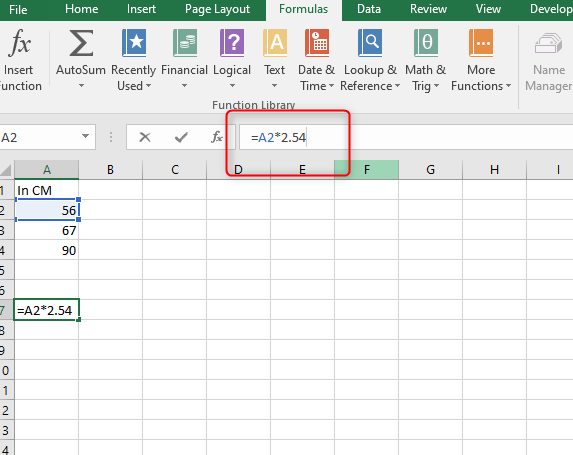# How to convert CM to inches in Excel

Microsoft Excel offers powerful conversion functionality to the user. In this way, one can easily convert cm to inches without any difficulty. It offers features that you can apply simple equations across ranges of data to quickly produce new figures. It only applies to; Microsoft ®Excel® 2010,2013,2016,2019 and 365(windows). Here's a detailed way of how you can achieve your goals of converting cm to inches or vice versa:

First and foremost, the basic information that you need to have at the back of your mind is:

1 inch=2.54cm

1cm=0.3937 inches.

After recognizing that, you'll then create a new spreadsheet in Microsoft Excel. To avoid confusion, how you create the spreadsheet is when you select "New" from the file menu, which will then provide you with the new spreadsheet, or by pressing " Ctrl + N " which will also provide you with the new spreadsheet as required. You'll then follow the below instructions carefully so that you can achieve your goals of converting cm to inches or vice versa.

## Ways on Converting Cm to INCHES

• Dividing to convert from cm to inches.
• Multiply to convert inches to cm.
• Using the convert function to facilitate the conversion.

## DIVIDING TO CONVERT FROM CM TO INCHES.

To convert your work from cm to inches by dividing follow the below steps:

1. Click the cell where you want to insert the converted amount.2. Key in =cell to convert /2.54 for example, ( =B2/2.54).3. Press the enter button.## MULTIPLY TO CONVERT INCHES TO CM.

To convert your work from inches to cm by multiplying follow the below steps:

1. Click the cell where you want to insert the converted amount.2. Key in =cell to convert ×2.54 for example,( =B2×2.54)3. Press the enter button.

## USING THE CONVERT FUNCTION.

You can also use the convert function to convert measurements. So, basically, the convert function applies this syntax: = convert ( cell to convert, units to convert from, units to convert to). What should be clearly noted, is that the units must be in the quotation or double marks for example ( "cm").

## CONVERTING CM TO INCHES USING THE CONVERT FUNCTION.

To convert your work from cm to Inches by using the convert function, follow these basic steps:

1. Click in a cell where you want to insert the converted amount.2. Key in = convert ( cell to convert, "cm", "in" for example, type = convert ( B2,"cm","in").3. Lastly, press enters to get your results.

## CONVERTING INCHES TO CM USING THE CONVERT FUNCTION.

To convert inches to cm by using the convert function, follow the following listed instructions:

1. Click in a cell where you want to insert the converted amount.2. Key in = convert ( cell to convert,"in","cm") for example, type = convert (B2,"in","cm").3. Last but not least, press the enter button to get your feedback.

NOTE: The convert function is so wide, it's not only limited to cm and inches conversion but also to other units, thus, this is very helpful to anyone who will require any form of help.